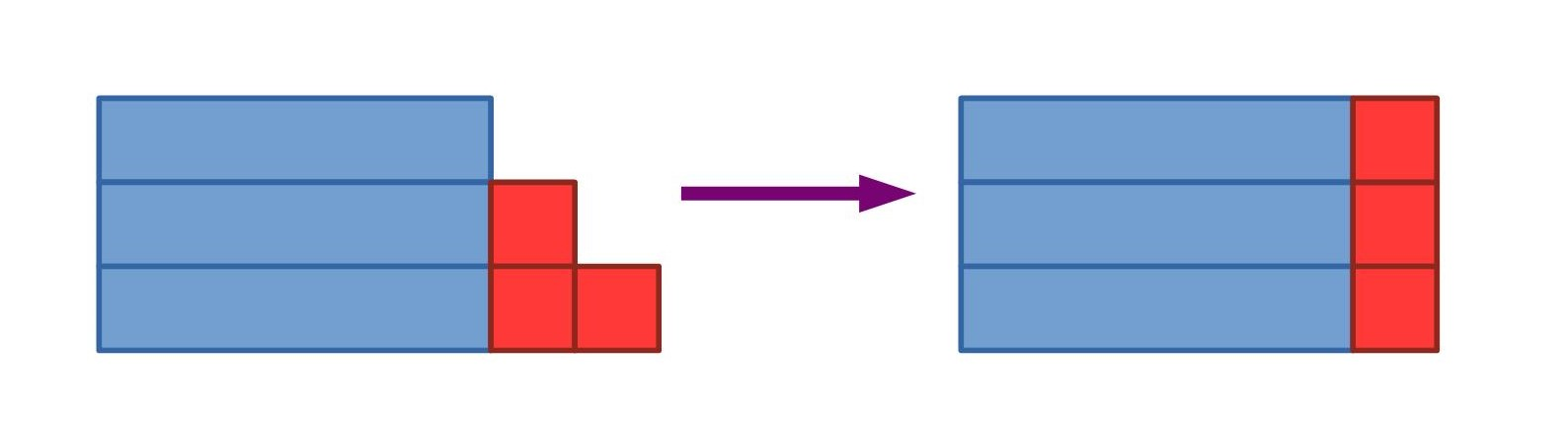#### You may also like### DOTS Division

Take any pair of two digit numbers x=ab and y=cd where, without loss of generality, ab > cd . Form two 4 digit numbers r=abcd and s=cdab and calculate: {r^2 - s^2} /{x^2 - y^2}.### Novemberish

a) A four digit number (in base 10) aabb is a perfect square. Discuss ways of systematically finding this number. (b) Prove that 11^{10}-1 is divisible by 100.### Latin Numbers

Can you create a Latin Square from multiples of a six digit number?

# Number Rules - OK

### Why do this problem?

In this problem students have the opportunity of taking the important mathematical step of starting from particular numerical cases and moving towards generalisations. After exploring each of the cases, testing numerical examples, students are challenged to provide visual and/or algebraic justifications for the results.

### Possible approach

This printable worksheet may be useful: Number Rules - OK

This activity featured in an NRICH Secondary webinar in March 2021.

Start by asking students to choose three consecutive numbers, and add them together.
Write a selection of students' responses on the board.
"What do you notice?" The answers are all multiples of 3.
"Will this always happen? Can you explain why?"

Give students some time to discuss with their partner why the answers are always multiples of 3. Circulate and listen out for interesting insights.

Bring the class together and share any explanations they have found. Perhaps share the following two representations if they haven't emerged:

Method 1:Method 2:

$$n+(n+1) + (n+2) = 3n + 3 = 3(n+1)$$

"These methods can be used to explain lots of similar rules. Here are a few more to explore. With your partner, try some numerical examples to convince yourself that each statement is true, and then try to provide convincing pictorial and/or algebraic arguments that they are always true."

While the class is working, circulate round the room looking out for pairs who have developed convincing arguments. When appropriate, bring the class together to share their explanations, or ask them to present their explanations on a poster to display.

### Key questions

Can you express the sum of two consecutive numbers algebraically?  Can you draw a picture (or bar diagram) to show the sum of two consecutive numbers?

How can you express an even number algebraically?  How about an odd number?

How can you express a multiple of $4$ algebraically?

How can you express an even number which is not a multiple of $4$ algebraically?

### Possible support

Summing Consecutive Numbers and Always a Multiple? might be good problems to prepare students for this one.

### Possible extension

Challenge students to discover other number rules and provide convincing arguments that they are always true.

Always Perfect might be a suitable follow-up activity.